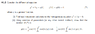# Variation of Parameters to solve a second order ODE

Bonnie

## Homework Statement

The question I am working on is the one in the file attached.

## Homework Equations

y = u1y1 + u2y2 :

u1'y1 + u2'y2 = 0
u1'y1' + u2'y2' = g(t)

## The Attempt at a Solution

I think I have got part (i) completed, with y1 = e3it and y2 = e-3it. This gives a general solution to the homogeneous equation to be y = C1cos3t + C2sin3t.
For part (ii) I know that for variation of parameters you need to substitute y1 and y2 and their derivatives into the above system of equations to solve for u1' and u2', then integrate these to find u1 and u2, from which you can get the desired solution of
y = yp + yh
But I find that when I try to do that by substituting y1 = e3it and y2 = e-3it I just end up with very complicated expressions involving lots of e's and i's which the desired solution does not contain. I know it involves combining the information about the general soln from (i) with the method I have described, but I just don't know how to make it work. Any help would be appreciated, thank you in advance!

#### Attachments

•Capture.PNG
10.5 KB · Views: 325

Homework Helper
I just end up with very complicated expressions involving lots of e's and i's which the desired solution does not contain
So the thing to do is avoid these exponential ##y## and take the trigonometric ##y## that you see in front of you when you write ##y = C_1\cos 3t + C_2\sin3t\ ## !

http://tutorial.math.lamar.edu/Classes/DE/VariationofParameters.aspx

Homework Helper
Dearly Missed

## Homework Statement

The question I am working on is the one in the file attached.

## Homework Equations

y = u1y1 + u2y2 :

u1'y1 + u2'y2 = 0
u1'y1' + u2'y2' = g(t)

## The Attempt at a Solution

I think I have got part (i) completed, with y1 = e3it and y2 = e-3it. This gives a general solution to the homogeneous equation to be y = C1cos3t + C2sin3t.
For part (ii) I know that for variation of parameters you need to substitute y1 and y2 and their derivatives into the above system of equations to solve for u1' and u2', then integrate these to find u1 and u2, from which you can get the desired solution of
y = yp + yh
But I find that when I try to do that by substituting y1 = e3it and y2 = e-3it I just end up with very complicated expressions involving lots of e's and i's which the desired solution does not contain. I know it involves combining the information about the general soln from (i) with the method I have described, but I just don't know how to make it work. Any help would be appreciated, thank you in advance!
Have you forgotten that ##e^{\pm i \theta} = \cos \theta \pm i \sin \theta \,?##

Bonnie
Have you forgotten that ##e^{\pm i \theta} = \cos \theta \pm i \sin \theta \,?##
Yes I had, thank you. I'll have another try!